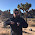Monday, September 19, 2016

Computers are stupid; they do exactly what we tell them to do. Excel spreadsheets don't make mistakes. I do. Like when I accidentally overwrote a number in a cell a few weeks ago. And that's not just in Excel. I have told computers to do stupid things with data in SAS, Visual Basic, Stata, Rats, R, etc. ... See a pattern? It's me. It's us. Not our algorithms.
That's from Claudia Sahm. Actually if you follow the link to Dave Giles blog (and then the first link Giles says to read), you will find out that: no, spreadsheets really do make mistakes. Here's an abstract from a paper Giles cites:
This paper discusses the numerical precision of five spreadsheets (Calc, Excel, Gnumeric, NeoOffice and Oleo) running on two hardware platforms (i386 and amd64) and on three operating systems (Windows Vista, Ubuntu Intrepid and Mac OS Leopard). The methodology consists of checking the number of correct significant digits returned by each spreadsheet when computing the sample mean, standard deviation, first-order autocorrelation, F statistic in ANOVA tests, linear and nonlinear regression and distribution functions. A discussion about the algorithms for pseudorandom number generation provided by these platforms is also conducted. We conclude that there is no safe choice among the spreadsheets here assessed: they all fail in nonlinear regression and they are not suited for Monte Carlo experiments.
This is not input errors, but rather actual errors in the software. Now it is true that the computers were told to do stupid things by the programmers of Excel, &c and as a general philosophy, human error is the source of lots of problems.

But really -- Excel computes things incorrectly even if you program it perfectly.

Now it is probably millions of times more likely that a given error you find is an error you yourself made, but every system out there has issues. Even Mathematica, my go-to math software (as you can probably tell from my graphs), has some bugs [pdf].

As always, the solution is to be alert and test results.

1.My numerical Lin algebra class had lots of good examples... E.g. don't compute a matrix exponential via the straight forward way it's definition suggests. Well, unless it's a 1x1 or converges 100% after a few terms.

1.Also the prof left us with a challenge: if we ever found a reason to compute a matrix inverse on its own to let him know. He'd never seen one.

2.Nor I. I've always needed it multiplied by something else.

3.Also, never form a Gramian if you can avoid it: expensive & you could lose half your sig figs.

4.I'd agree, however I think the findings are not that poor methods were frequently chosen (some cases), but that there are full-on mistakes in the code. E.g. the random number generator is implemented incorrectly.

2.O/T: have you seen this?
1.